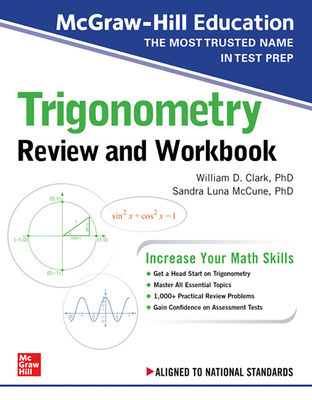## Digital Products

Connect®
Course managementreporting, and student learning tools backed by great support.

Connect® Master
Next Level Learning for Today's Generation

McGraw Hill GO
Greenlight learning with the new eBook+

ALEKS®
Personalize learning and assessment

ALEKS® Placement, Preparation, and Learning
Achieve accurate math placement

SIMnet
Ignite mastery of MS Office and IT skills

Get learning that fits anytime, anywhere

Sharpen: Study App
A reliable study app for students

## Services

Inclusive Access
Reduce costs and increase success

LMS Integration

Math Placement
Achieve accurate math placement

Curate and deliver your ideal content

Custom Courseware Solutions

Professional Services
Collaborate to optimize outcomes

Lecture Capture
Capture lectures for anytime access

Remote Proctoring
Validate online exams even offsite

Institutional Solutions
Increase engagement, lower costs, and improve access for your students

#### Support

General Help & Support Info
Customer Service & Tech Support contact information

Online Technical Support Center
FAQs, articles, chat, email or phone support

Support At Every Step
Instructor tools, training and resources for ALEKS, Connect & SIMnet

Instructor Sample Requests
Get step by step instructions for requesting an evaluation, exam, or desk copy

Platform System Check
System status in real time# McGraw-Hill Education Trigonometry Review and Workbook, 1st Edition

ISBN10: 126012892X | ISBN13: 9781260128925
By William Clark and Sandra Luna McCune

## Purchase Options:

* The estimated amount of time this product will be on the market is based on a number of factors, including faculty input to instructional design and the prior revision cycle and updates to academic research-which typically results in a revision cycle ranging from every two to four years for this product. Pricing subject to change at any time.

## Instructor Details

This engaging review guide and workbook is the ideal tool for sharpening your Trigonometry skills!

This review guide and workbook will help you strengthen your Trigonometry knowledge, and it will enable you to develop new math skills to excel in your high school classwork and on standardized tests. Clear and concise explanations will walk you step by step through each essential math concept. 500 practical review questions, in turn, provide extensive opportunities for you to practice your new skills. If you are looking for material based on national or state standards, this book is your ideal study tool!

Features:

Aligned to national standards, including the Common Core State Standards, as well as the standards of non-Common Core states and Canada
Designed to help you excel in the classroom and on standardized tests
Concise, clear explanations offer step-by-step instruction so you can easily grasp key concepts
You will learn how to apply Trigonometry to practical situations
500 review questions provide extensive opportunities for you to practice what you’ve learned

Introduction
CHAPTER 1 Angles and Their Measure
Definitions and Terminology
Complementary and Supplementary Angles
Coterminal Angles and Reference Angles
CHAPTER 2 Concepts from Geometry
The Sum of a Triangle’s Angles and the Triangle Inequality
The Pythagorean Theorem
CHAPTER 3 Right Triangle Trigonometry
Trigonometric Ratios of an Acute Angle in a Right Triangle
Trigonometric Ratios of Special Acute Angles
CHAPTER 4 General Right Triangles
Solving Right Triangles
Applications of Right Triangle Trigonometry
CHAPTER 5 Oblique Triangles
Law of Cosines (SAS or SSS)
Law of Sines (ASA or AAS)
Law of Sines Ambiguous Case (SSA)
Solving General Triangles
Area of a General Triangle Using Trigonometry
CHAPTER 6 Trigonometric Functions of Any Angle
Definitions of the Trigonometric Functions
Trigonometric Functions of Complementary Angles
The Unit Circle
Trigonometric Functions of Coterminal Angles
Trigonometric Functions of Negative Angles
Using Reference Angles to Find the Values of Trigonometric Functions
CHAPTER 7 Trigonometric Identities
Definition and Guidelines
The Reciprocal and Ratio Identities
The Pythagorean Identities
Sum and Difference Formulas for the Sine Function
Sum and Difference Formulas for the Cosine Function
Sum and Difference Formulas for the Tangent Function
Reduction Formulas
Double-Angle Identities
Half-Angle Identities
Sum-to-Product Identities
Product-to-Sum Identities
CHAPTER 8 Trigonometric Functions of Real Numbers
Definitions and Basic Concepts of Trigonometric Functions of Real Numbers
Periodic Functions
CHAPTER 9 Graphs of the Sine Function
The Graph of y = sin x
The Graph of y = A sin x
The Graph of y = A sin Bx
The Graph of y = A sin (Bx – C)
The Graph of y = A sin (Bx – C) + D
CHAPTER 10 Graphs of the Cosine Function
The Graph of y = cos x
The Graph of y = A cos (Bx – C) + D
CHAPTER 11 Graphs of the Tangent Function
The Graph of y = tan x
The Graph of y = A tan (Bx – C) + D
CHAPTER 12 Graphs of the Secant, Cosecant, and Cotangent Functions
The Graph of y = A sec (Bx – C) + D
The Graph of y = A csc (Bx – C) + D
The Graph of y = A cot (Bx – C) + D
CHAPTER 13 Inverse Trigonometric Functions
The Inverse Sine, Cosine, and Tangent Functions
The Inverse Secant, Cosecant, and Cotangent Functions
CHAPTER 14 Solving Trigonometric Equations
Basic Concepts of Trigonometric Equations
Solving for Exact Solutions to Trigonometric Equations
Solving for Approximate Solutions to Trigonometric Equations
CHAPTER 15 Trigonometric Form of a Complex Number
Definition of the Trigonometric Form of a Complex Number
The Product and Quotient of Trigonometric Forms of Complex Numbers
De Moivre’s Theorem
Roots of Complex Numbers
CHAPTER 16 Polar Coordinates
Basic Concepts of Polar Coordinates
Converting Between Coordinate Systems
Graphing Equations in Polar Form
Glossary
APPENDIX A Calculator Instructions for Trigonometry Using the TI-84 Plus
General Usage
Setting the Calculator to Degree or Radian Mode
Evaluating Trigonometric Functions
Determining Inverse Trigonometric Values
Graphing Polar Equations
APPENDIX B Trigonometric Identities
APPENDIX C The Complex Plane

William Clark

Sandra Luna McCune

Need support?   We're here to help - Get real-world support and resources every step of the way.